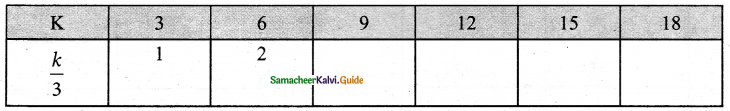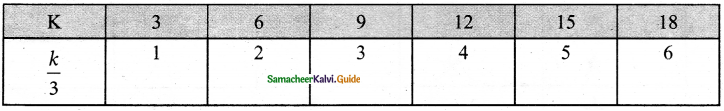Students can download Maths Chapter 2 Introduction to Algebra Ex 2.2 Questions and Answers, Notes, Samacheer Kalvi 6th Maths Guide Pdf helps you to revise the complete Tamilnadu State Board New Syllabus, helps students complete homework assignments and to score high marks in board exams.

Tamilnadu Samacheer Kalvi 6th Maths Solutions Term 1 Chapter 2 Introduction to Algebra Ex 2.2

Question 1.
Find in the blanks.
(i) The algebraic statement of ‘f’ decreased by 5 is _______
(ii) The algebraic statement of ‘s’ divided by 5 is _______
(iii) The verbal statement of ‘2m – 10’ is _______
(iv) If A’s age is ‘n’ years now, 7 years ago A’s age was ______
(v) If ‘p – 5’ gives 12 then ‘p’ is ______
Solution:
(i) f – 5
(ii) $$\frac { s }{ 5 }$$
(iii) 10 less than 2 times m (or) Take away 10 from the product of 2 and m
(iv) n – 7
(v) 17
Hint: p – 5 = 12 ⇒ n = 12 + 5 = 17Question 2.
Say True or False.
(i) 10 more to three times ‘c’ is ‘3c + 13’.
(ii) If the cost of 10 rice bags is ‘t’, then the cost of 1 rice bag is $$\frac { t }{ 10 }$$
(iii) The statements ‘x’ divided by 3 and 3 divided by ‘x’ are the same.
(iv) The product of ‘q’ and 20 is ’20q’
(v) 7 less to 7 times ‘y’ is ‘7 – 7y’
Solution:
(i) False
Hint: 3c + 10
(ii) True
(iii) False
(iv) True
(v) False
Hint: 7y – 7Question 3.
Express the following verbal statement to an algebraic statement.
(i) ‘t’ is added to 100
(ii) 4 times ‘q’
(iii) 8 is reduced by ‘y’
(iv) 56 added to 2 times ‘x’
(v) 4 less to 9 times of ‘y’
Solution:
(i) t + 100
(ii) 4q
(iii) 8 – y
(iv) 2x + 56
(v) 9y – 4Question 4.
Express the following algebraic statement to a verbal statement.
(i) x ÷ 3
(ii) 5n – 12
(iii) 11 + 10x
(iv) 70s
Solution:
(i) x divided by 3.
(ii) 12 less to 5 times n.
(iii) 11 added to 10 times x
(iv) 7 times s.Question 5.
The teacher asked two students to write the algebraic statement for the verbal statement “8 more than a number”. Vetri wrote ‘8 + x’ but Maran wrote ‘8x’. Who gave the correct answer?
Solution:
Let the number be x; 8 more than the number = 8 + x.
Vetri gave the correct answer as 8 + x.

Question 6.
(i) If ‘n’ takes the value 3 then find the value of ‘n + 10’?
(ii) If ‘g’ is equal to 300 what is the value of ‘g – 1’ and ‘g + 1’?
(iii) What is the value of ‘s’, if ‘2s – 6’ gives 30?
Solution:
(i) n = 3
n + 10 = 3 + 10 = 13(ii) g = 300
g – 1 = 300 – 1
= 299
g + 1 = 300 + 1
=301

(iii) 2s – 6 = 30
2s = 30 + 6
2s = 36
s = 36/2
s = 18Question 7.
Complete the table and find the value of ‘k’ for which ‘k/3’ gives 5.Solution:$$\frac{k}{3}$$ = 5
k = 15

Question 8.
The value of ‘y’ in y + 7 = 13 is
(a) y = 5
(b) y = 6
(c) y = 7
(d) y = 8
Solution:
(b) y = 6
Hint: y = 13 – 7 = 6Question 9.
6 less to ‘n’ gives 8 is represented as
(a) n – 6 = 8
(b) 6 – n = 8
(c) 8 – n = 6
(d) n – 8 = 6
Solution:
(a) n – 6 = 8

Question 10.
The value of ‘c’ for which $$\frac{3c}{4}$$ gives 18 is
(a) c = 15
(b) c = 21
(c) c = 24
(d) c = 27
Solution:
(c) c = 24